Open in App
Not now

# Class 12 RD Sharma Solutions – Chapter 30 Linear Programming – Exercise 30.2 | Set 2

• Last Updated : 20 May, 2021

### Question 11. Minimize Z = 5x + 3y

Subject to

2x + y ≥ 10

x + 3y ≥ 15

x ≤ 10

y ≤ 8

x, y ≥ 0

Solution:

Convert the given in equations into equations, we will get the following equations:

2x + y = 10, x + 3y = 15, x = 10, y = 8

Area shown by 2x + y ≥ 10:

The line 2x + y = 10 meets the coordinate axes at A(5, 0) and B(0, 10) respectively.

After connecting these points we will get the line 2x + y = 10.

Thus,

(0,0) does not assure the in equation 2x + y ≥ 10.

Thus,

The area in xy plane which does not have the origin represents the solution set of the in equation 2x + y ≥ 10.

The area represented by x + 3y ≥ 15:

The line x + 3y = 15 connects the coordinate axes at C(15, 0) and D(0, 5) respectively.

After connecting these points we will get the line x + 3y = 15.

Thus,

(0,0) assure the in equation x + 3y ≥ 15.

The area in xy plane which does not have the origin represents the solution set of the in equation x + 3y ≥ 15.

The line x = 10 is the line that passes through the point (10, 0) and is parallel to Y axis.x ≤ 10 is the area to the left of the line

x = 10.

The line y = 8 is the line that passes through the point (0, 8) and is parallel to X axis.y ≤ 8 is the area below the line y = 8.

The area shows by x ≥ 0 and y ≥ 0:

Thus,

All point in the first quadrant assure these in equations.

Thus,

The first quadrant is the area shows by the in equations x ≥ 0 and y ≥ 0.

The suitable area determined by the system of constraints, 2x + y ≥ 10, x + 3y ≥ 15, x ≤ 10, y ≤ 8, x ≥ 0 and y ≥ 0 are as

follows.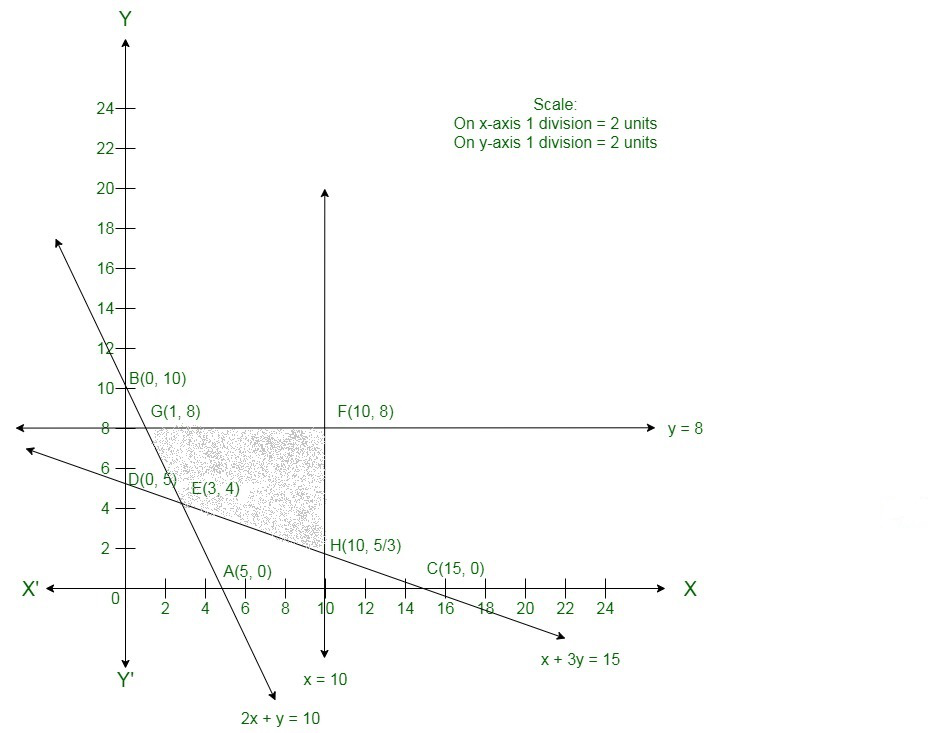The corner points of the suitable region are

E(3, 4),

HF(10, 8) and

G(1, 8).

The values of Z at these corner points are as follows.

Hence,

The minimum value of Z is 27 at the point F(3, 4).

Therefore,

x = 3 and y =4 is the best solution of the given LPP.

Hence, the best value of Z is 27.

### Question 12.  Minimize Z = 30x + 20y

Subject to

x + y ≤ 8

x + 4y ≥ 12

5x + 8y = 20

x, y ≥ 0

Solution:

Convert the given in equations into equations, we will get the following equations:

x + y = 8, x + 4y = 12, x = 0 and y = 0

5x + 8y = 20 is already an equation.

The area shows by x + y ≤ 8:

The line x + y = 8 connects the coordinate axes at A(8, 0) and B(0, 8) respectively.

After connecting these points we will get the line x + y = 8.

Thus,

(0,0) assures the in equation x + y ≤ 8.

Thus,

The area in xy plane which have the origin represents the solution set of the in equation x + y ≤ 8.

The area shows by x + 4y ≥ 12:

The line x + 4y = 12 connects the coordinate axes at C(12, 0) and D(0, 3) respectively.

After connecting these points we will get the line x + 4y = 12.

Thus,

(0,0) assure the in equation x + 4y ≥ 12.

Thus,

The area in xy plane which does not have the origin shows the solution set of the in equation x + 4y ≥ 12.

The line 5x + 8y = 20 is the line that passes through E(4, 0) and F.

The area shows by x ≥ 0 and y ≥ 0:

Thus,

All point in the first quadrant assure these in equations.

Thus,

The first quadrant is the area shows by the in equations x ≥ 0 and y ≥ 0.

The suitable area determined by the system of constraints, x + y ≤ 8, x + 4y ≥ 12, 5x + 8y = 20, x ≥ 0 and y ≥ 0 are as follows.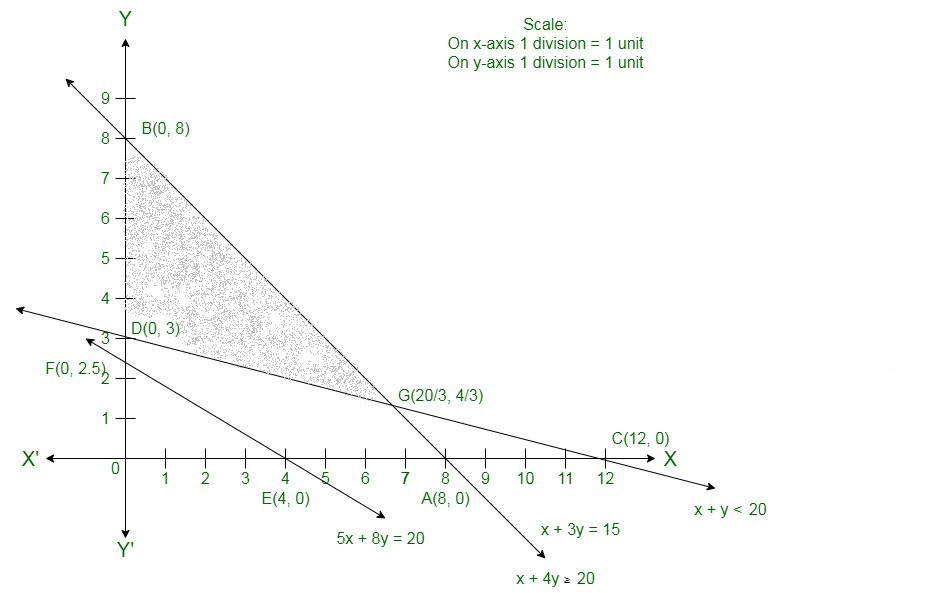The corner points of the suitable area are B(0,8), D(0,3), G.
The values of Z at these corner points are as follows.

Hence,

The minimum value of Z is 60 at the point D(0,3).

Therefore,

x = 0 and y =3 is the best solution of the given LPP.

Therefore,

The best value of Z is 60.

### Question 13.  Maximize Z = 4x + 3y

Subject to

3x + 4y ≤ 24

8x + 6y ≤ 48

x ≤ 6000

x ≤ y

x, y ≥ 0

Solution:

Here, we need to maximize Z = 4x + 3y

Convert the given in equations into equations, we will get the following equations:

3x + 4y = 24, 8x + 6y = 48, x = 5 , y = 6, x = 0 and y = 0.

The line 3x + 4y = 24 connects the coordinate axis at A(8, 0) and B(0,6).

Connects these points to get the line 3x + 4y = 24.

Thus,

(0, 0) assure the in equation 3x + 4y ≤ 24.

Thus,

The area in xy-plane that have the origin represents the solution set of the given equation.

The line 8x + 6y = 48 connects the coordinate axis at C(6, 0) and D(0,8).

Connect these points to get the line 8x + 6y = 48.

Thus,

(0, 0) assure the in equation 8x + 6y  ≤ 48.

Thus,

The area in xy-plane that have the origin represents the solution set of the given equation.

x = 5 is the line passing through x = 5 parallel to the Y axis.

y = 6 is the line passing through y = 6 parallel to the X axis.

The area shown by x ≥ 0 and y ≥ 0:

Hence,

All the points in the first quadrant assure these in equations.

Thus,

The first quadrant is the area shown by the in equations.

These lines are drawn using a suitable scale.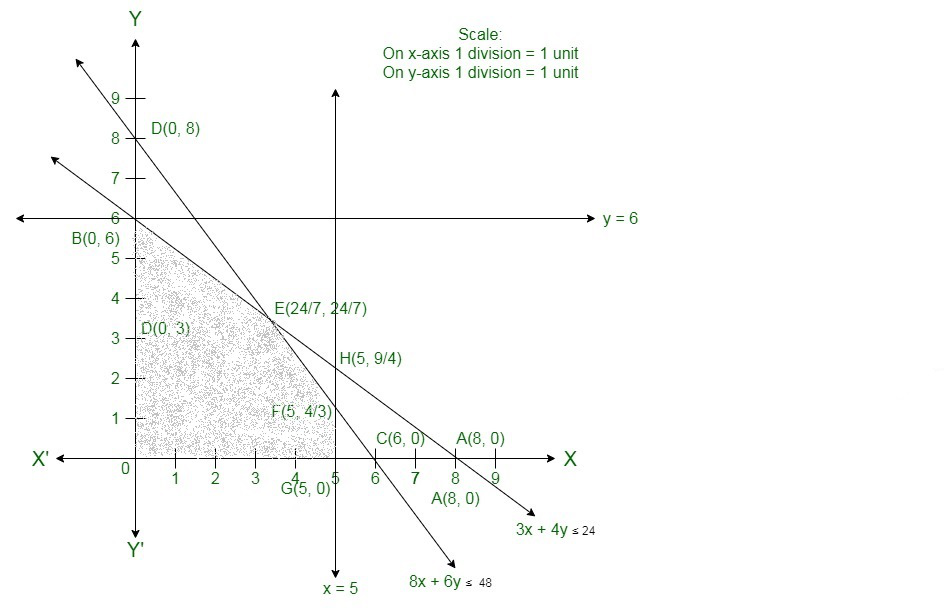The corner points of the feasible region are O(0, 0), G(5, 0), F, Eand B(0, 6).

The values of Z at these corner points are as follows.

Here we can see that the maximum value of the objective function Z is 24 which is at Fand E.

Therefore,

The best value of Z is 24.

### Question 14. Minimize Z = x – 5y + 20

Subject to

x – y ≥ 0

-x + 2y ≥ 2

x ≥ 3

y ≤  4

x, y ≥ 0

Solution:

Convert the given in equations into equations, we will get the following equations:

x − y = 0, − x + 2y = 2, x = 3, y = 4, x = 0 and y = 0.

The area shown by x − y ≥ 0 or x ≥ y:

The line x − y = 0 or x = y passes through the origin.The area to the right of the line x = y will assure the given in equation.

Now we will check by taking an example like if we take a point (4, 3) to the right of the line x = y .

Here, x ≥ y.

Thus,

It assure the given in equation.

Take a point (4, 5) to the left of the line x = y. Here, x ≤ y. That means it does not assure the given in equation.

The area shown by − x + 2y ≥ 2:

The line − x + 2y = 2 connects the coordinate axes at A(−2, 0) and B(0, 1) respectively.

After connecting these points we will get the line − x + 2y = 2.

Thus,

(0,0) does not assure the in equation − x + 2y ≥ 2.

Thus,

The area in xy plane which does not have the origin represents the solution set of the in equation − x + 2y ≥ 2 .

The line x = 3 is the line that passes through the point (3, 0) and is parallel to Y axis. x ≥ 3 is the area to the right of the

line x = 3.

The line y = 4 is the line that passes through the point (0, 4) and is parallel to X axis. y ≤ 4 is the area below the line y = 4.

The area shown by x ≥ 0 and y ≥ 0:

Hence,

All point in the first quadrant assure these in equations.

Thus, the first quadrant is the area shown by the in equations x ≥ 0 and y ≥ 0.

The suitable area determined by the system of constraints x − y ≥ 0,− x + 2y ≥ 2, x ≥ 3, y ≤ 4, x ≥ 0 and y ≥ 0 are as follows.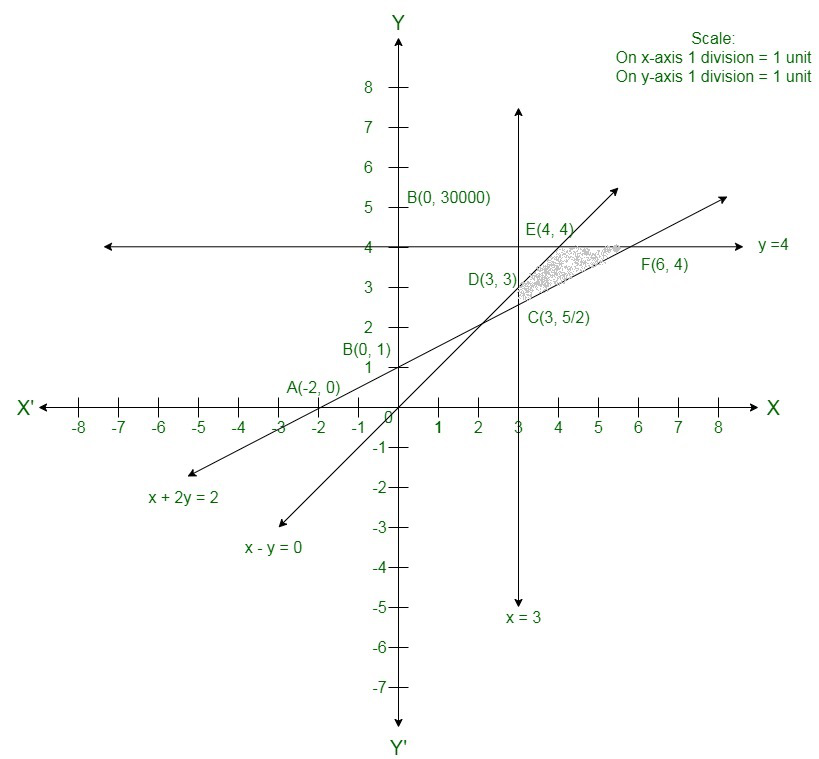The corner points of the suitable area are C, D(3, 3), E(4, 4) and F(6, 4).

The values of Z at these corner points are as follows.

Hence,

The minimum value of Z is 4 at the point E(4, 4).

Therefore,

x = 4 and y = 4 is the best solution of the given LPP.

Hence,

The best value of Z is 4.

### Question 15.  Maximize Z = 3x + 5y

Subject to

x + 2y ≤ 20

x + y ≤ 15

y ≤ 15

x, y ≥ 0

Solution:

Here we need to maximize Z = 3x + 5y

Convert the given in equations into equations, we will get the following equations:

x + 2y = 20, x + y = 15, y = 5, x = 0 and y = 0.

The line x + 2y = 20 connects the coordinate axis at A(20, 0) and B(0,10).

Connect these points to get the line x + 2y = 20.

Thus,

(0, 0) assume the in equation x + 2y ≤ 20.

Thus,

The area in xy-plane that have the origin represents the solution set of the given equation.

The line x + y = 15 connect the coordinate axis at C(15, 0) and D(0,15).

Connect these points to get the line x + y = 15.

Thus,

(0, 0) assume the in equation x + y ≤ 15.

Thus,

The area in xy-plane that have the origin represents the solution set of the given equation.

y = 5 is the line passing through (0, 5) and parallel to the X axis. The area below the line y = 5 will assure the given in equation.

The area shown by x ≥ 0 and y ≥ 0:

Hence,

All the point in the first quadrant assure these in equations.

Thus,

The first quadrant is the area shown by the in equations.

These lines are drawn using a suitable scale.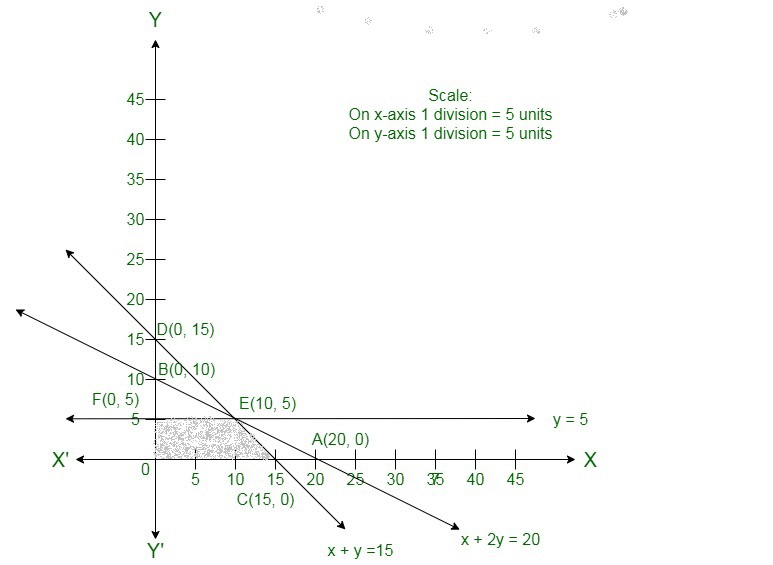The corner points of the suitable area are O(0, 0), C(15, 0), E(10, 5) and F(0, 5)

The values of Z at these corner points are as follows.

Here we can see that the maximum value of the objective function Z is 55 which is at E(10, 5).

Therefore,

The best value of Z is 55.

### Question 16. Minimize Z = 3x1 + 5x2

Subject to

x1 + x2 ≥ 3

x1 + x2 ≥ 2

x1, x2 ≥ 0

Solution:

Convert the given in equations into equations, we will get the following equations:

x1 + 3x2 = 3, x1 + x2 = 2, x1 = 0 and x2 = 0

The area shown by x1 + 3x2 ≥ 3 :

The line x1 + 3x2 = 3 connects the coordinate axes at A(3, 0) and B(0, 1) respectively.

After connecting these points we will get the line x1 + 3x2 = 3.

Thus,

(0,0) does not assure the in equation x1 + 3x2 ≥ 3.

Thus,

The area in the plane which does not have the origin represents the solution set of the in equation x1 + 3x2 ≥ 3.

The area shown by x1 + x2 ≥ 2:

The line x1 + x2 = 2 connects the coordinate axes at C(2, 0) and D(0, 2) respectively.

After connecting these points we will get the line x1 + x2 = 2.

Thus,

(0,0) does not assure the in equation x1 + x2 ≥ 2.

Thus,

The area having the origin represents the solution set of the in equation x1 + x2 ≥ 2.

The area shown by x1 ≥ 0 and x2 ≥ 0:

Hence,

All the points in the first quadrant assure these in equations.

Thus,

The first quadrant is the area shown by the in equations x1 ≥ 0 and x2 ≥ 0.

The suitable area determined by the system of constraints, x1 + 3x2 ≥ 3 , x1 + x2 ≥ 2, x1 ≥ 0, and x2 ≥ 0, are as follows.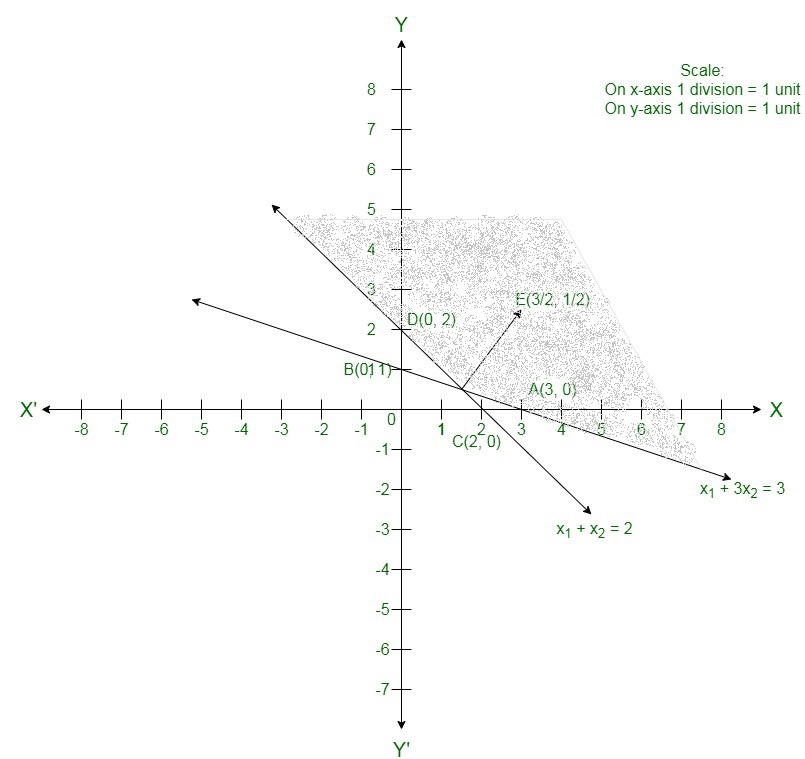The corner points of the suitable area are O(0, 0), B(0, 1), Eand C(2, 0).

The values of Z at these corner points are as follows.

Hence,

The minimum value of Z is 0 at the point O(0, 0).

Hence,

x1 = 0 and x2 = 0 is the best solution of the given LPP.

Therefore,

The best value of Z is 0.

### Question 17.  Maximize Z = 2x + 3y

Subject to

x + y ≥ 1

10x + y ≥ 5

x + 10y ≥ 1

x, y ≥ 0

Solution:

Convert the given in equations into equations, we will get the following equations:

x + y = 1, 10x +y = 5, x + 10y = 1, x = 0 and y = 0

The area shown by x + y ≥ 1:

The line x + y = 1 connects the coordinate axes at A(1, 0) and B(0,1) respectively.

After connecting these points we will get the line x + y = 1.

Thus,

(0,0) does not assure the in equation x + y ≥ 1.

Thus,

The area in xy plane which does not have the origin represents the solution set of the in equation x + y ≥ 1.

The area shown by 10x +y ≥ 5:

The line 10x +y = 5 connect the coordinate axes at Cand D(0, 5) respectively.

After connecting these points we will get the line 10x +y = 5.

Thus,

(0,0) does not assure the in equation 10x +y ≥ 5.

Thus, the area which does not have the origin represents the solution set of the in equation 10x +y ≥ 5.

The area shown by x + 10y ≥ 1:

The line x + 10y = 1 connect the coordinate axes at A(1, 0) and Frespectively.

After connecting these points we will have the line x + 10y = 1.

Thus,

(0,0) does not assure the in equation x + 10y ≥ 1.

Thus, the area which does not have the origin represents the solution set of the in equation x + 10y ≥ 1.

The area shown by x ≥ 0 and y ≥ 0:

Here,

All the points in the first quadrant assure these in equations.

Thus,

The first quadrant is the area shown by the in equations x ≥ 0, and y ≥ 0.

The suitable area determined by the system of constraints x + y ≥ 1, 10x +y ≥ 5, x + 10y ≥ 1, x ≥ 0, and y ≥ 0, are as follows.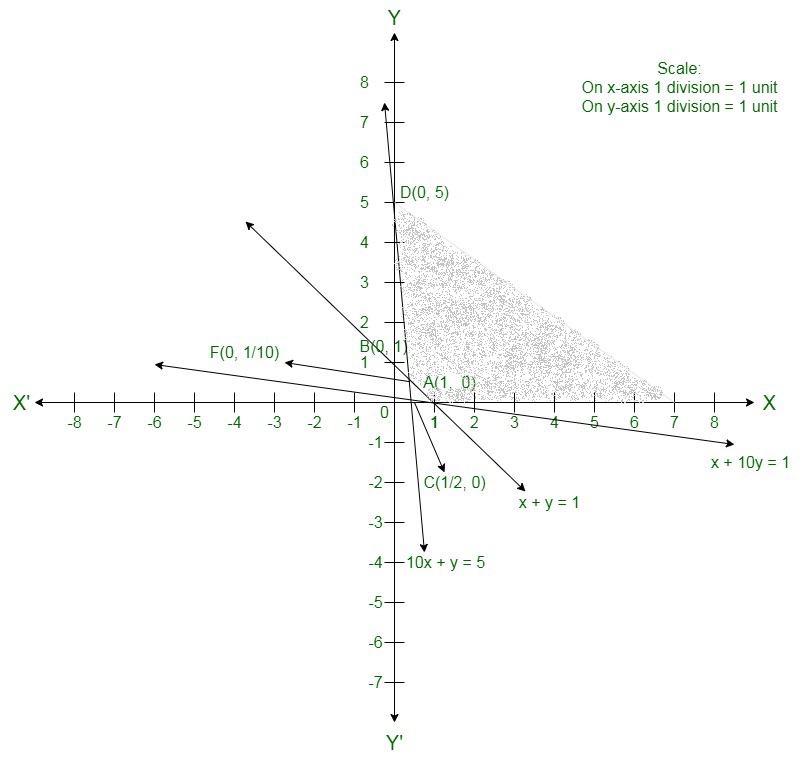The suitable area is unbounded.

Hence,

The maximum value is infinity i.e. the solution is unbounded.

### Question 18. Maximize Z = -x1 + 2x2

Subject to

-x1 + 3x2 ≤ 10

x1 + x2 ≤ 6

x1 – x2 ≤ 2

x1, x2 ≥ 0

Solution:

Convert the given in equations into equations, we will get the following equations:

−x1 + 3x2 = 10, x1 + x2 = 6, x1 + x2 = 2, x1 = 0 and x2 = 0

The area shown by −x1 + 3x2 ≤ 10:

The line −x1 + 3x2 = 10 coincide the coordinate axes at A(−10, 0) and Brespectively.

After connecting these points we will get the line −x1 + 3x2 = 10.

Thus,

(0,0) satisfies the in equation −x1 + 3x2 ≤ 10 .

Thus,

The area region in the plane which have the origin shows the solution set of the in equation

−x1 + 3x2 ≤ 10.

The area shown by x1 + x2 ≤ 6:

The line x1 + x2 = 6 connects the coordinate axes at C(6, 0) and D(0, 6) respectively.

After connecting these points we will get the line x1 + x2 = 6.

Thus,

(0,0) assure the in equation x1 + x2 ≤ 6.

Thus,

The area having the origin represents the solution set of the in equation x1 + x2 ≤ 6.

The area shown by x1− x2 ≤ 2:

The line x1 − x2 = 2 coincide the coordinate axes at E(2, 0) and F(0, −2) respectively.

After connecting these points we will get the line x1 − x2 = 2.

Thus,

(0,0) assure the in equation x1− x2 ≤ 2.

Thus,

The area having the origin represents the solution set of the in equation x1− x2 ≤ 2.

The area shown by x1 ≥ 0 and x2 ≥ 0:

Hence,

All the points in the first quadrant assure these in equations.

Thus,

The first quadrant is the region shown by the in equations x1 ≥ 0 and x2 ≥ 0.

The suitable area determined by the system of constraints, −x1 + 3x2 ≤ 10, x1 + x2 ≤ 6, x1− x2 ≤ 2, x1 ≥ 0, and x2 ≥ 0, are as follows.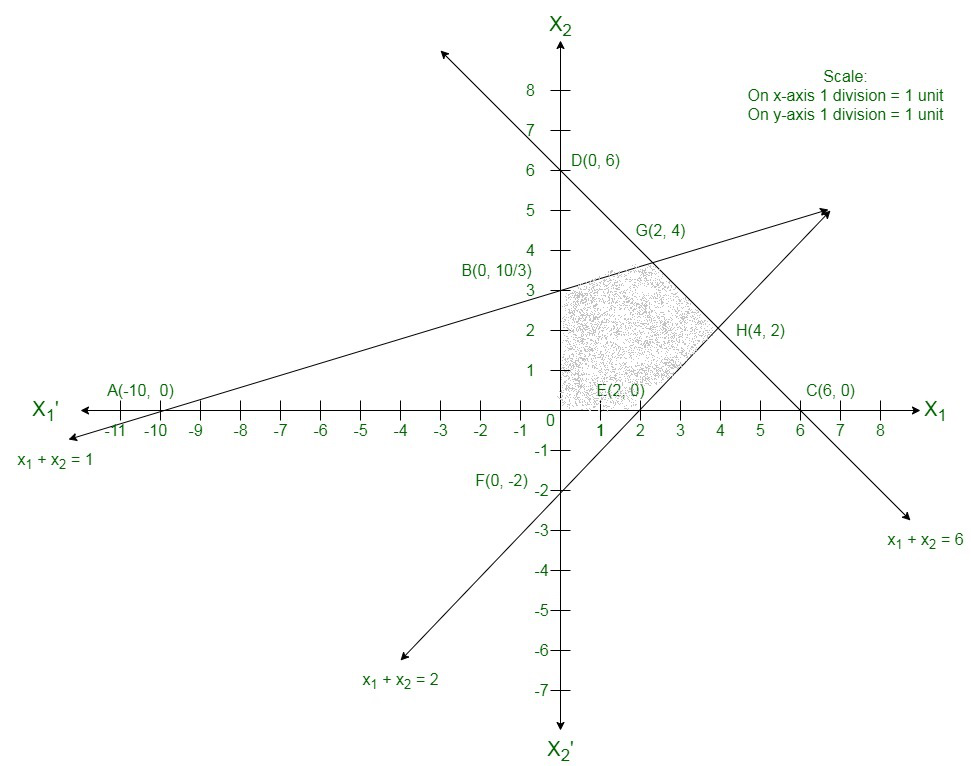The corner points of the assure area are O(0, 0), E(2, 0), H(4, 2), G(2, 4) and B.

The values of Z at these corner points are as follows.

Here we can see that the maximum value of the objective function Z iswhich is at B.

### Question 19. Maximize Z = -x + y

Subject to

-2x + y ≤ 1

x  ≤ 2

x + y ≤ 3

x, x ≥ 0

Solution:

Here we need to maximize Z = x + y

Convert the given in equations into equations, we will get the following equations:

−2x + y = 1, x = 2, x + y = 3, x = 0 and y = 0.

The line −2x + y = 1 coincide the coordinate axis at Aand B(0, 1).

Now connect these points to get the line −2x + y = 1 .

Thus,

(0, 0) assure the in equation −2x + y ≤ 1.

Thus,

The area in xy-plane that have the origin represents the solution set of the given equation.

x = 2 is the line passing through (2, 0) and parallel to the Y axis.

The area below the line x = 2 will assure the given in equation.

The line x + y = 3 coincide the coordinate axis at C(3, 0) and D(0, 3).

Connect these points to get the line x + y = 3.

Thus,

(0, 0) assure the in equation x + y ≤ 3.

Thus,

The area in xy-plane that have the origin shows the solution set of the given equation.

The area shown by x ≥ 0 and y ≥ 0:

Hence,

All the points in the first quadrant assures these in equations.

Thus,

The first quadrant is the area shown by the in equations.

These lines are drawn using a satisfactory scale.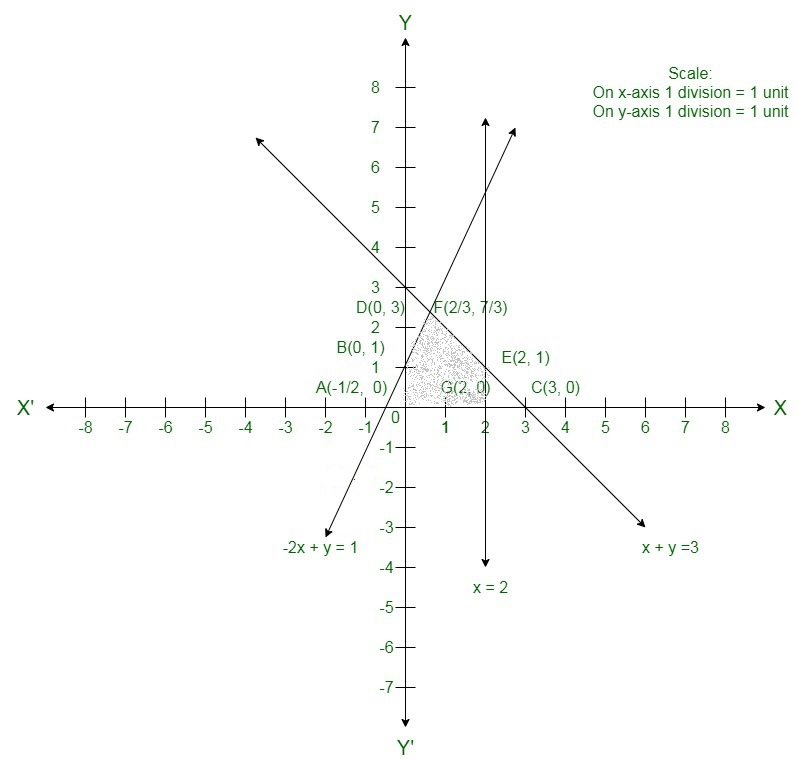The corner points of the suitable area are O(0, 0),G(2, 0), E(2, 1) and FThe values of Z at these corner points are as follows.

Here we can see that the maximum value of the objective function Z is 3 which is at E(2, 1) and F.

Therefore,

The best value of Z is 3.

### Question 20. Maximize Z = -3x1 + 4x2

Subject to

x1 – x2 ≤ -1

x1 + x2  ≤ 0

x1, x2 ≥ 0

Solution:

Convert the given in equations into equations, we will get the following equations:

x1 − x2 = −1, −x1 + x2 = 0, x1 = 0 and x2 = 0

The area shown by x1 − x2 ≤ −1:

The line x1 − x2 = −1 coincide the coordinate axes at A(−1, 0) and B(0, 1) respectively.

After connecting these points we will get the line x1 − x2 = −1.

Thus,

(0,0) does not assures the in equation x1 − x2 ≤ −1 .

Thus,

The area in the plane which does not have the origin shows the solution set of the in equation x1 − x2 ≤ −1.

The area shown by −x1 + x2 ≤ 0 or x1 ≥ x2:

The line −x1 + x2 = 0 or x1 = x2 is the line passing through (0, 0).

The area to the right of the line x1 = x2 will assure the given in equation −x1 + x2 ≤ 0.

If we mark a point (1, 3) to the left of the line x1 = x2

Here, 1≤3 which is not assuring the in equation x1 ≥ x2

Hence,

The area to the right of the line x1 = x2 will assure the given in equation −x1 + x2 ≤ 0.

The area shown by x1 ≥ 0 and x2 ≥ 0:

Thus,

All the points in the first quadrant assure these in equations.

Thus,

The first quadrant is the area shown by the in equations x1 ≥ 0 and x2 ≥ 0.

The suitable area determined by the system of constraints, x1 − x2 ≤ −1, −x1 + x2 ≤ 0, x1 ≥ 0, and x2 ≥ 0, are as follows.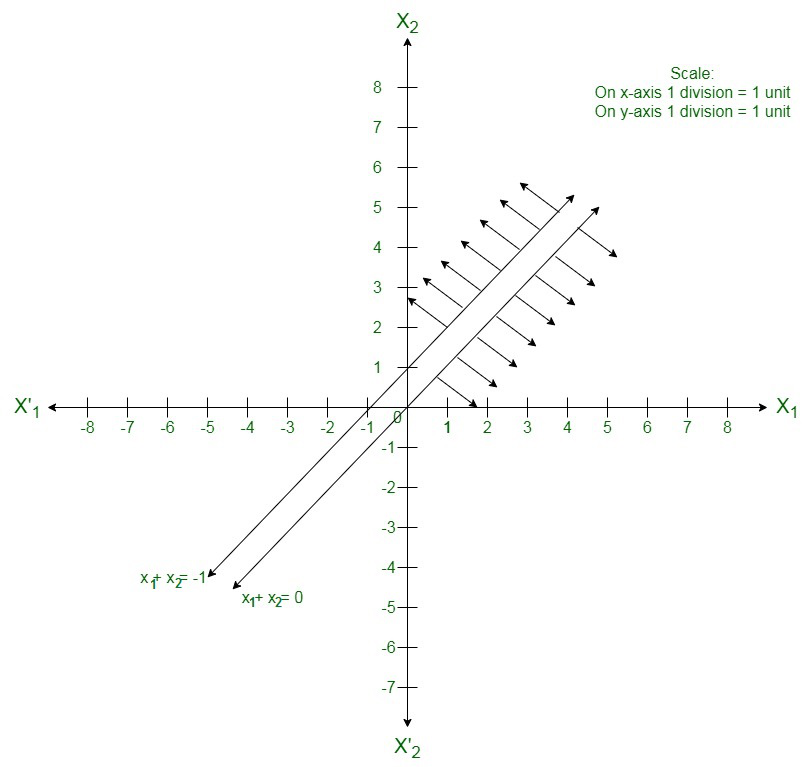Here we can see that the suitable area of the given LPP does not exist.

My Personal Notes arrow_drop_up